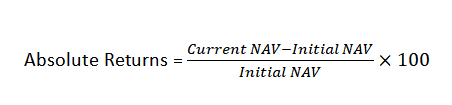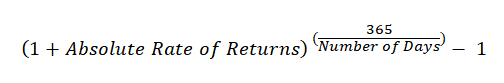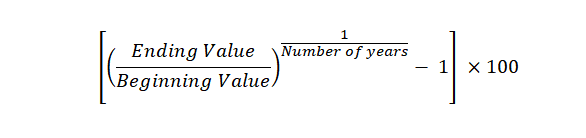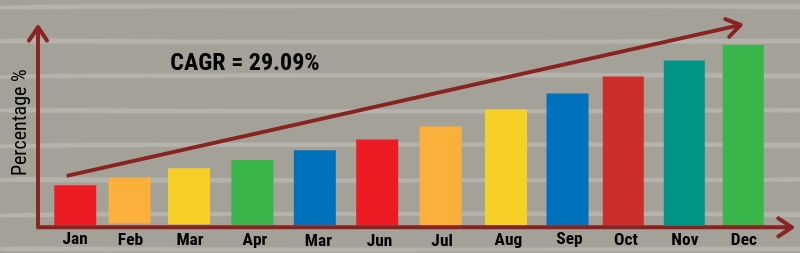ARTICLESHave you invested in Mutual Funds? What has been your ROI (Return on Investment)? How do you calculate mutual fund returns? Do you think this Corona Crisis affecting your mutual fund returns?

Give us a few minutes of your precious time and read this piece; You will learn, how to calculate mutual fund returns with worked-out examples and formula for calculating different types of mutual fund returns.

You will also get to know how to calculate the profit percentage in the mutual fund for investments done for different periods.

## Mutual Fund Returns and Corona Virus Market Crash

This article just deals with basic mutual fund returns calculations. If you need info on that please proceed further.

• Why my mutual fund returns are low now?
• Is it ok to come out or need to stay invested…because I have got poor or negative returns?
• How to take advantage of this corona crisis to recover my financial life?

Then, You may read the below article

Attention Investors! Want To Take Unfair Advantage of the CoronaVirus Crash? You Are Just One Step Away

• As my mutual fund returns are low because of the market crash, what should I do now to increase my mutual fund returns?
• How can I recover my mutual fund portfolio faster and better?
• How to use my Mutual Fund SIP to recover my portfolio from the market crash?

Then, you may read the below article

How To Make Your Portfolio Recover Better & Faster From the Stock Market Crash? (Part 1)

HHow To Make Your Portfolio Recover Better & Faster From The Stock Market Crash—Part-2: Discover The Rewarding Nature of Portfolio Rebalancing

HHow To Make Your Portfolio Recover Better & Faster From The Stock Market Crash—Part-3: Play Smart with Your SIP: Stop or Continue or Increase?

## What is the ‘return’ on a mutual fund scheme?

In simple terms, ‘return’ is the yield that your investment generates over a period of time. It is the percentage increase or decrease in the value of the investment in that period.

#### The simple ways to calculate the returns are given below:

We will discuss them in detail. But first, let’s understand, what is the significance of Net Asset Value (NAV) in Mutual Fund calculations.

## So, what is NAV?

According to SEBI, the performance of a particular scheme of a mutual fund is denoted by Net Asset Value (NAV). NAV is the market value of the securities held by the scheme. Since the market value of securities changes every day, NAV of a scheme also varies on day to day basis.

The NAV per unit is the market value of securities of a scheme divided by the total number of units of the scheme on any particular date.

Purchase NAV and sale NAV are two important numbers for mutual fund profit calculation.

#### Example: How to calculate NAV of mutual fund

If the market value of securities of a mutual fund scheme is Rs 300 lakhs and the mutual fund has issued 20 lakh units of Rs. 10 each to the investors, then the NAV per unit of the fund is Rs.15.

i.e. 300 Lakhs / 20 Lakhs = 15

To know more about NAV, you can read this detailed post on Net Asset Value (NAV).

## 1. Absolute Returns

This method is common when the holding period of your investment is less than 12 months. It helps you calculate the simple returns on your initial investment.

Absolute Returns refers to the returns that a fund achieves over a period of time.It measures the percentage appreciation or depreciation in the value of the NAV over a certain time frame.

To calculate absolute returns, all you need is the current NAV and the initial NAV of your investment.
Simply put,#### Example: Mutual Fund Return on Investment Calculation

If the current NAV is 15 and the previous NAV was 13.5,

the return would be (15 – 13.5) x 100/13.5 = 150/13.5 = 11.11% over the time period.

If the time period is in months say 3 months or in years say 2, or in days say 100, in that case, the above formula can be used asThe above example will produce returns of 11.11 x 4 = 44.44% (for 3 months); or 5.55% for 2 years or 40.55% for a period of 100 days.

#### Example 2: Calculation of the absolute returns of a Mutual Fund:

If you have purchased it at Rs.11 per unit and after 3 years, if NAV appreciates to Rs. 15 per unit, here the absolute return is 36.36% as calculated below:

(15 – 11) x 100 / 11 = 36.36%

## 2. Simple Annualized ReturnsThis method is used when the investment duration is exactly 1 year.

The formula to find out the simple annualized returns is given as:You may put this in the excel sheet to calculate it.

For example, the NAV of Rs. 20 may shoot to Rs. 25 in the next 8 months, that is, 240 days. The absolute returns can be calculated as (25-20)/20 = 0.25.

To find the simple annualized returns, we use the formula described above,

(1 + 0.25)^(365/240) – 1 = 40.4%

## 3. CAGR (Compounded Annual Growth Rate)

When the investment duration is more than 1 year, CAGR is a better way to depict returns. The value of CAGR indicates that how the investment would have grown had it generated a steady return.

The CAGR returns are annualized returns, with the compounding effect.

The formula used to find CAGR is given below,#### Example: CAGR Calculation

The purchase NAV of your MF is Rs.15 per unit. After two years NAV rises to Rs.25.

Then CAGR will be 29.09%

i.e. [(25/15)^(1/2) -1].

The CAGR calculates the growth rate of investment every year with the compounding effect. In the above example, in case your investment was Rs1500 which has appreciated by 29.09% each year to become Rs.2500 at the end of two years.## Absolute Returns Vs Annualized Returns

When you are calculating returns for less than a year, you can calculate absolute return. For calculating mutual fund returns for an investment period of more than a year then you can use annualised returns.

When you need to calculate point to point returns, you can use absolute return. When you want to calculate the average yearly return, then you can use annualised return.

## 4. XIRR (for calculating SIP Returns)

XIRR is a function in Excel for calculating the Internal Rate of Returns for an array of cash flows occurring at an irregular interval. To calculate XIRR, you need the data given below

• SIP Amount
• Dates of SIP investments
• Date of redemption; and
• Maturity (Redemption) Amount

Let’s take an example:

Suppose you have invested Rs. 2000 every month for the last 1 year and the value of your investment rose to Rs. 26000 due to appreciation in NAV. The following table illustrates your SIP investment:

## Returns on SIP (Systematic Investment Plans)

Suppose you have invested Rs. 2000 every month for the last 1 year and value of your investment rose to Rs. 26000 due to appreciation in NAV. The following table illustrates your SIP investment:

 Total Amount Invested Jan 1, 2019 2000 Feb 1, 2019 2000 Mar 1, 2019 2000 Apr 1, 2019 2000 May 1, 2019 2000 Jun 1, 2019 2000 Jul 1, 2019 2000 Aug 1, 2019 2000 Sep 1, 2019 2000 Oct 1, 2019 2000 Nov 1, 2019 2000 Dec 1, 2019 2000 Jan 1, 2020 Rs. 26000 XIRR 15.65% Rs. 24000

As the investor invests Rs.2000 per month for 1 year, the absolute returns formula will not work as the money is invested for different periods of time.

The IRR (Internal Rate of return) considers the time value of money for investment made at different point of time. Therefore, we may use XIRR returns (which is nothing but IRR) in MS Excel to find out the return on SIP in the above example, which is 15.65%

## 5. Mutual Fund SIP calculator to build a corpus

The above 4 formulae helps you in calculating the returns you have earned.

The below automated calculator will help you project and find how much will be the future value of your SIP investments.

This calculator also can be used to find out how much you need to save to accumulate a particular corpus.

## Final Words

You now know very simple ways to calculate returns on Mutual Fund investments. To know the NAVs of MF schemes, you may have to go to the website of AMFI (The Association of Mutual Funds in India) at www.amfiindia.com.

Have you tried any other way to calculate mutual fund returns? What result did you get? How much time did it consume? Kindly share your views in the comment box.

If you are REALLY inclined to make this solid difference in achieving your financial goals by creating a financial plan, then I would suggest you to test-drive our services by opting for## One thought on “How to Calculate Mutual Fund Returns”

1.Manivel says:

Thanks a lot!